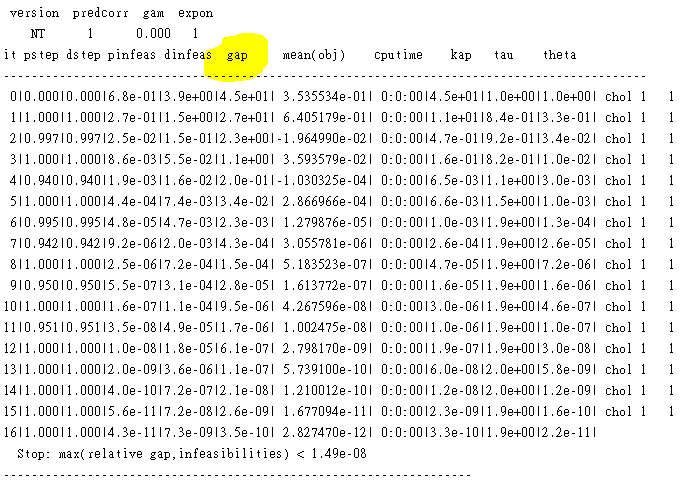# How can I set the print output of cvx to a variable in matlab?

Hi everybody,

How can I set the print output of cvx to a new variable in matlab?

Thanks very much.

Can you please describe more clearly what you want to do?

Thanks for your commets. The following are my code and output. I wan to assign the output to a new variable.

cvx_begin
variables x(2) y;
subject to
{x,y} == lorentz(2);
B1
[x’,y]’<=[0;0];
cvx_end

## Calling SDPT3 4.0: 10 variables, 4 equality constraints For improved efficiency, SDPT3 is solving the dual problem.

num. of constraints = 4
dim. of socp var = 8, num. of socp blk = 2
dim. of linear var = 2

SDPT3: Infeasible path-following algorithms

## number of iterations = 10 primal objective value = -3.16478366e+00 dual objective value = -3.16478367e+00 gap := trace(XZ) = 2.50e-09 relative gap = 3.41e-10 actual relative gap = 3.39e-10 rel. primal infeas (scaled problem) = 1.46e-13 rel. dual " " " = 2.24e-12 rel. primal infeas (unscaled problem) = 0.00e+00 rel. dual " " " = 0.00e+00 norm(X), norm(y), norm(Z) = 6.5e+00, 5.8e-01, 8.2e-01 norm(A), norm(b), norm© = 4.5e+00, 9.0e+00, 2.0e+00 Total CPU time (secs) = 0.09 CPU time per iteration = 0.01 termination code = 0 DIMACS: 1.7e-13 0.0e+00 2.2e-12 0.0e+00 3.4e-10 3.4e-10

Status: Solved
Optimal value (cvx_optval): -0.16855

After the solver finishes and CVX exits, the CVX variables become MATLAB variables in the workspace having the optimal values So x and y will have the optimal values just computed. cvx_optval will have the optimal objective value. You can use these as you would any other MATLAB variables. You can of course assign a new MATLAB variable (or array element) to equal any of these if you so desire. If you invoke CVX again with these same variable names as CVX variables, they will be overwritten with the values from the new run, as will cvx_optval.

Hi I want to express x(1)^2 + x(2)^2=y^2 as a constraint in the cvx. How can I do that?

My code is as follows. But It can not run.

U=[ 2.0647, 0 , -1.8435; 0 , 0.9080 , 0;-1.8435, 0, 1.6475]
w=mvnrnd([0;0;0],inv(Q),1)’;
b=-2Qw;
p=B0’b;
cvx_begin quiet
variables x(2) y;
subject to
norm(x)==norm(y);
B1
[x’,y]’<=[0;0];
cvx_end
cvx_x=B0
[x’,y]’;

This is a non-convex nonlinear constraint, and is not allowed by CVX.

Dear Mark,

I have another question similar to this question.
How can I set the gap to the matlab variable?Do you want to record the final gap in a MATLAB variable? If so, the only thing I can think of is using the MATLAB diary command to record the displayed output, then extract the gap from the diary file using a batch editor, script, or program, for instance in PERL called via MATLAB system command…

If you want to control what the final gap can be, you need to do indirectly influence that with cvx_precision or via solver_settings to control solver options.